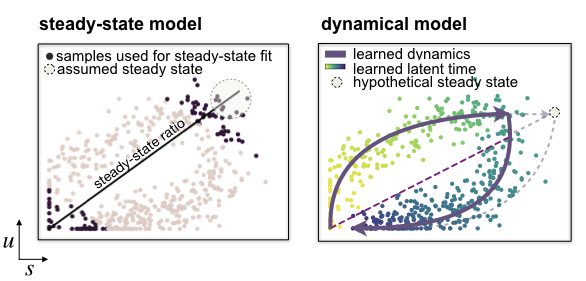# scvelo.tl.velocity¶

scvelo.tl.velocity(data, vkey='velocity', mode='stochastic', fit_offset=False, fit_offset2=False, filter_genes=False, groups=None, groupby=None, groups_for_fit=None, constrain_ratio=None, use_raw=False, use_latent_time=None, perc=[5, 95], min_r2=0.01, min_likelihood=0.001, r2_adjusted=None, use_highly_variable=True, diff_kinetics=None, copy=False, **kwargs)

Estimates velocities in a gene-specific manner.

The steady-state model [Manno18] determines velocities by quantifying how observations deviate from a presumed steady-state equilibrium ratio of unspliced to spliced mRNA levels. This steady-state ratio is obtained by performing a linear regression restricting the input data to the extreme quantiles. By including second-order moments, the stochastic model [Bergen19] exploits not only the balance of unspliced to spliced mRNA levels but also their covariation. By contrast, the likelihood-based dynamical model [Bergen19] solves the full splicing kinetics and generalizes RNA velocity estimation to transient systems. It is also capable of capturing non-observed steady states.Parameters: data : AnnData Annotated data matrix. vkey : str (default: ‘velocity’) Name under which to refer to the computed velocities for velocity_graph and velocity_embedding. mode : ‘deterministic’, ‘stochastic’ or ‘dynamical’ (default: ‘stochastic’) Whether to run the estimation using the steady-state/deterministic, stochastic or dynamical model of transcriptional dynamics. The dynamical model requires to run tl.recover_dynamics first. fit_offset : bool (default: False) Whether to fit with offset for first order moment dynamics. fit_offset2 : bool, (default: False) Whether to fit with offset for second order moment dynamics. filter_genes : bool (default: True) Whether to remove genes that are not used for further velocity analysis. groups : str, list (default: None) Subset of groups, e.g. [‘g1’, ‘g2’, ‘g3’], to which velocity analysis shall be restricted. groupby : str, list or np.ndarray (default: None) Key of observations grouping to consider. groups_for_fit : str, list or np.ndarray (default: None) Subset of groups, e.g. [‘g1’, ‘g2’, ‘g3’], to which steady-state fitting shall be restricted. constrain_ratio : float or tuple of type float or None: (default: None) Bounds for the steady-state ratio. use_raw : bool (default: False) Whether to use raw data for estimation. use_latent_time : boolor None (default: None) Whether to use latent time as a regularization for velocity estimation. perc : float (default: [5, 95]) Percentile, e.g. 98, for extreme quantile fit. min_r2 : float (default: 0.01) Minimum threshold for coefficient of determination min_likelihood : float (default: None) Minimal likelihood for velocity genes to fit the model on. r2_adjusted : bool (default: None) Whether to compute coefficient of determination on full data fit (adjusted) or extreme quantile fit (None) use_highly_variable : bool (default: True) Whether to use highly variable genes only, stored in .var[‘highly_variable’]. copy : bool (default: False) Return a copy instead of writing to adata. velocity (.layers) – velocity vectors for each individual cell velocity_genes, velocity_beta, velocity_gamma, velocity_r2 (.var) – parameters